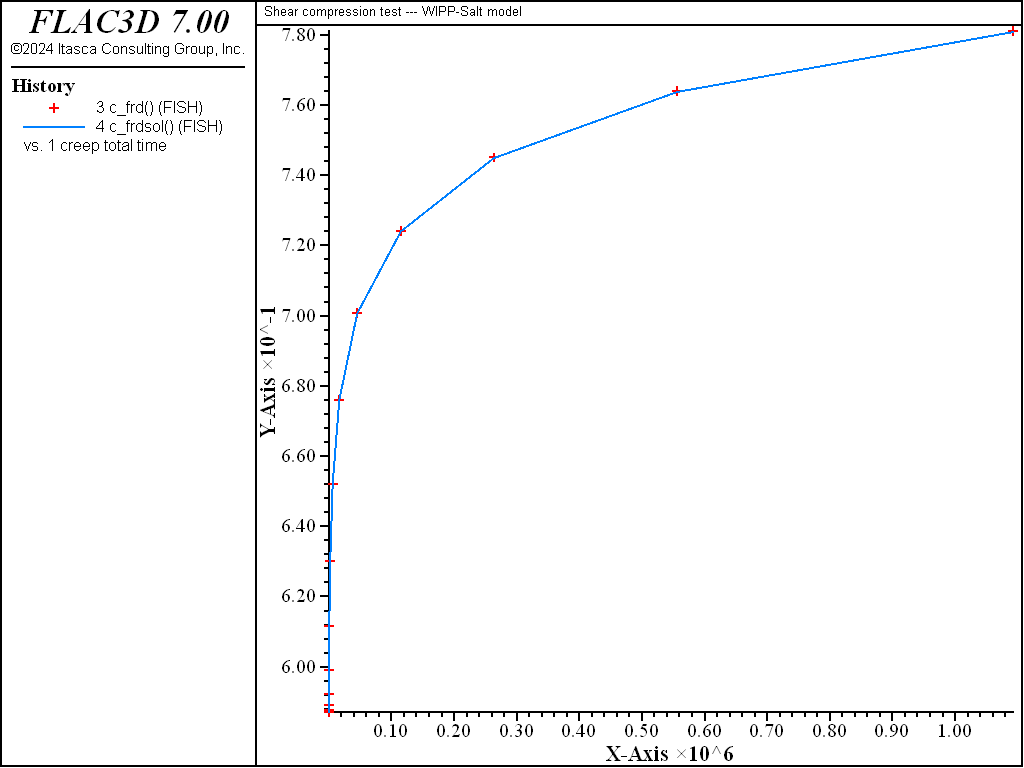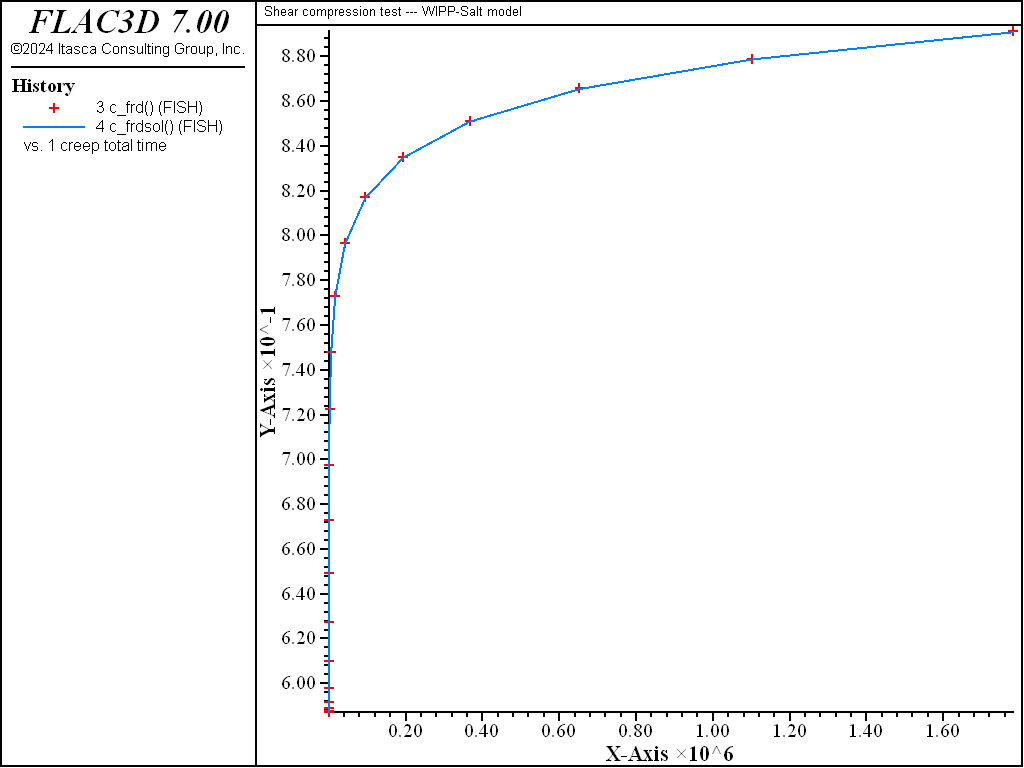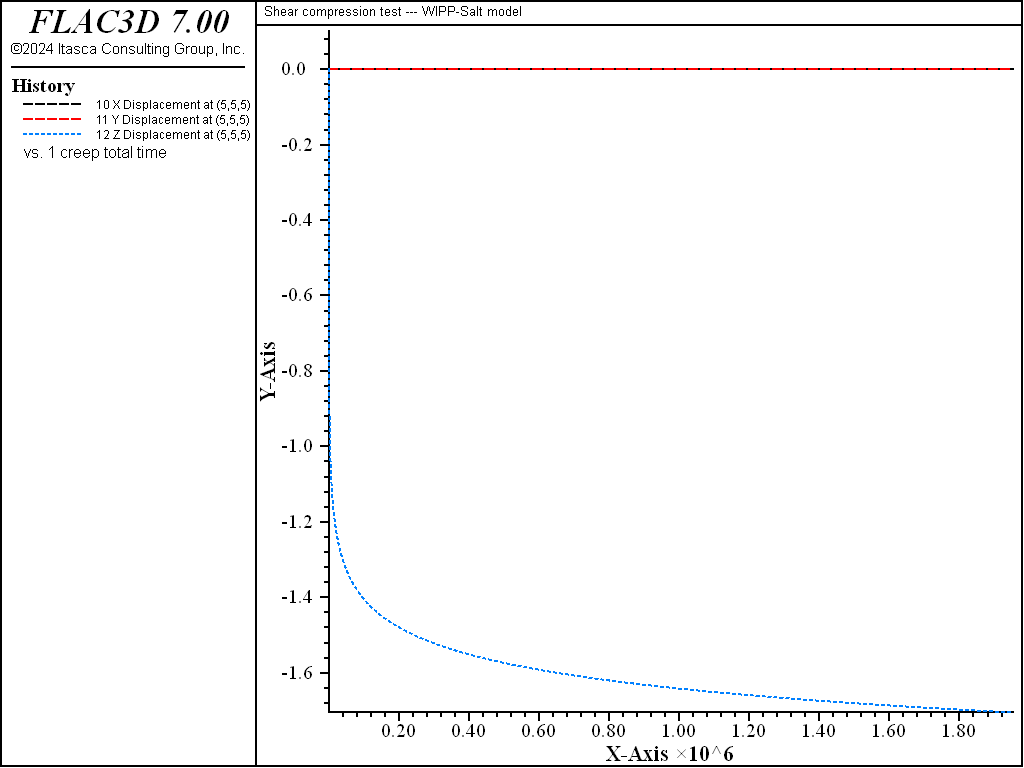# WIPP-Salt Model: Shear Compression Test

Note

To view this project in FLAC3D, use the menu command Help ► Examples…. Choose “Creep/ UnconfinedAndBiaxialCompressionWIPPSalt” and select “UnconfinedAndBiaxialCompressionWIPPSalt.prj” to load. The project’s main data files are shown at the end of this example.

Results of the shear compression test here are presented to validate the WIPP-Salt model in FLAC3D. The test involves one zone at an initially elastic state of equilibrium. The zone is allowed to creep for a period of 23 days, during which time the fractional density is monitored and compared to the analytical calculation.

In the shear compression test, both axial and confining stresses are kept constant. Using a notation convention in which $$\sigma_1$$ refers to the most negative (major) compressive stress and $$\sigma_3$$ refers to the least negative (minor) compressive stress, which is also the confining stress, then the stress invariants are

(1)$\sigma = {{1}\over{3}} (\sigma_1 + 2 \sigma_3)$

and

(2)$\bar\sigma = \sigma_3 - \sigma_1$

Also, we have

(3)$\sigma_{zz}^d = - {{2}\over{3}} (\sigma_3 - \sigma_1)$

and

(4)$\sigma_{xx}^d = \sigma_{yy}^d = {{1}\over{3}} (\sigma_3 - \sigma_1)$

For constant applied stresses, neglecting the creep component, the total strain rate equals the compaction strain rate, and we may write, using $$\beta$$ = 1 in the Equation on $$\dot\epsilon_{ij}^c$$ in WIPP-Salt model,

(5)$\dot\epsilon_{ij} = \dot\epsilon_v^c \left[{{\delta_{ij}}\over{3}} - {{\sigma_{ij}^d}\over{\bar{\sigma}}} \right]$

where $$\dot\epsilon_v^c$$ is given in WIPP-Salt model.

Substitution of Equation (1) to Equation (4) in Equation (5) yields

(6)$\dot{\epsilon_{zz}} = \dot{\epsilon_v^d}$
(7)$\dot{\epsilon_{xx}} = \dot{\epsilon_{yy}} = 0$

and no lateral compaction is predicted in this test for the constraints of the model.

The analytical expression for the fractional density is similar to that obtained for the hydrostatic compression test.

Two tests are performed: an unconfined compression test and a biaxial compression test. In both tests, $$\sigma_1$$ = -8.97 MPa. $$\sigma_3$$ = 0.0 in the unconfined compression test, and $$\sigma_3$$ = -6.90 MPa in the biaxial compression test. Initial and final density, and shear and bulk moduli are identical to those adopted in the hydrostatic compression test.

Numerical values of fractional density are compared to analytical values versus creep time for the unconfined compression test in Figure 1, and for the biaxial compression test in Figure 2. The error in fractional density is less than 0.1%. The histories of axial and lateral displacements for the unconfined compression test are shown in Figure 3, and for the biaxial compression test in Figure 4. As expected, no lateral displacement is calculated in these tests.Figure 1: Comparison of numerical and analytical prediction for fractional density for uniaxial compression.Figure 2: Comparison of numerical and analytical prediction for fractional density for biaxial compression.Figure 3: Histories of axial and lateral displacement for uniaxial compression.Figure 4: Histories of axial and lateral displacement for biaxial compression.

Data File

;------------------------------------------------------------------
;       Shear Compression test -- WIPP-Salt model
;-------------------------------------------------------------------
model new
fish automatic-create off
model title "Shear compression test --- WIPP-Salt model"
model configure creep
; --- geometry ---
zone create brick point 0 0 0 0 point 1 5 0 0 point 2 0 5 0 ...
point 3 0 0 5 size 1 1 1 ratio 1 1 1
; --- properties ---
zone initialize density 1350
program call 'parameter'
[cons]
; --- constitutive model ---
zone cmodel assign wipp-salt
zone property bulk 0.1186e9 shear 0.0714e9 activation-energy 12000 ...
constant-a 4.56 constant-b 127 constant-d 5.79e-36 ...
creep-rate-critical 5.39e-8 exponent 4.9 temperature 300 ...
constant-gas 1.987 bulk-final [valbf] shear-final [valsf] ...
density-final [valrhof] compaction-0 [valb0] ...
compaction-1 [valb1] compaction-2 [valb2] density-salt [valrho]

; --- histories ---
model history creep time-total
model history timestep
fish history c_frd
fish history c_frdsol
fish history c_err
fish history c_ev
fish history c_evsol
fish history c_rbulk
zone history displacement-x position 5 5 0
zone history displacement-x position 5 5 5
zone history displacement-y position 5 5 5
zone history displacement-z position 5 5 5
; --- test setup
model large-strain on
model creep active on
model creep timestep starting 5.e-5
model creep timestep min 5.e-5 max 5e4
; --- boundary conditions ---
zone gridpoint fix velocity-x range position-x 0
zone gridpoint fix velocity-y range position-y 0
zone gridpoint fix velocity-z range position-z 0
zone face apply stress-zz [valsig1] range position-z 5
; --- initial conditions ---
zone initialize stress-zz [valsig1]
model save 'ini'

; --- test uniaxial compression ---
[global valsig3=0.0] ; uniaxial compression
zone face apply stress-xx [valsig3] range position-x 5
zone face apply stress-yy [valsig3] range position-y 5
zone initialize stress-xx [valsig3]
zone initialize stress-yy [valsig3]
model solve time-total 2e6
model save 'uniaxial'

; --- test biaxial compression ---
model restore 'ini'
[global valsig3 = -6.90e6] ; biaxial compression
zone face apply stress-xx [valsig3] range position-x 5
zone face apply stress-yy [valsig3] range position-y 5
zone initialize stress-xx [valsig3]
zone initialize stress-yy [valsig3]
model solve time-total 2e6
model save 'biaxial'
;